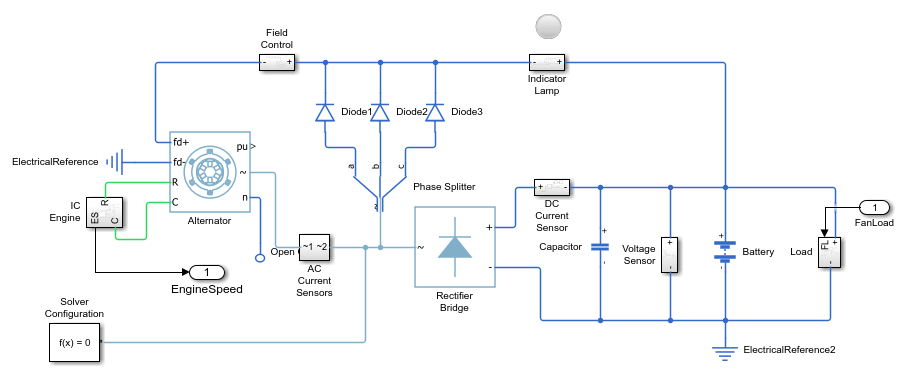# Vehicle Electrical and Climate Control Systems

This example shows how to model a vehicle climate control system with an electrical system. Use the model to examine the loading effects of the climate control system on the entire vehicle electrical system.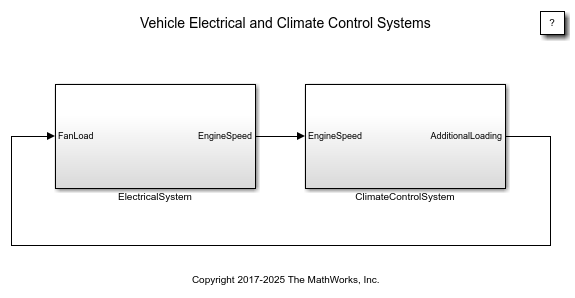### Climate Control System

To open the climate control system model, double-click the `ClimateControlSystem` subsystem. You can specify these settings:

• Internal vehicle temperature – Subsystem block named `UserSetpointInCelius` block. Enter a value for the temperature.

• External vehicle temperature – Subsystem block named `ExternalTemperatureInCelius`. Enter a value for the temperature.

The Display block named Thermometer Display provides the temperature reading of a sensor behind the head of the drive. As the model runs when the climate control is active, this reading indicates the temperature the driver feels.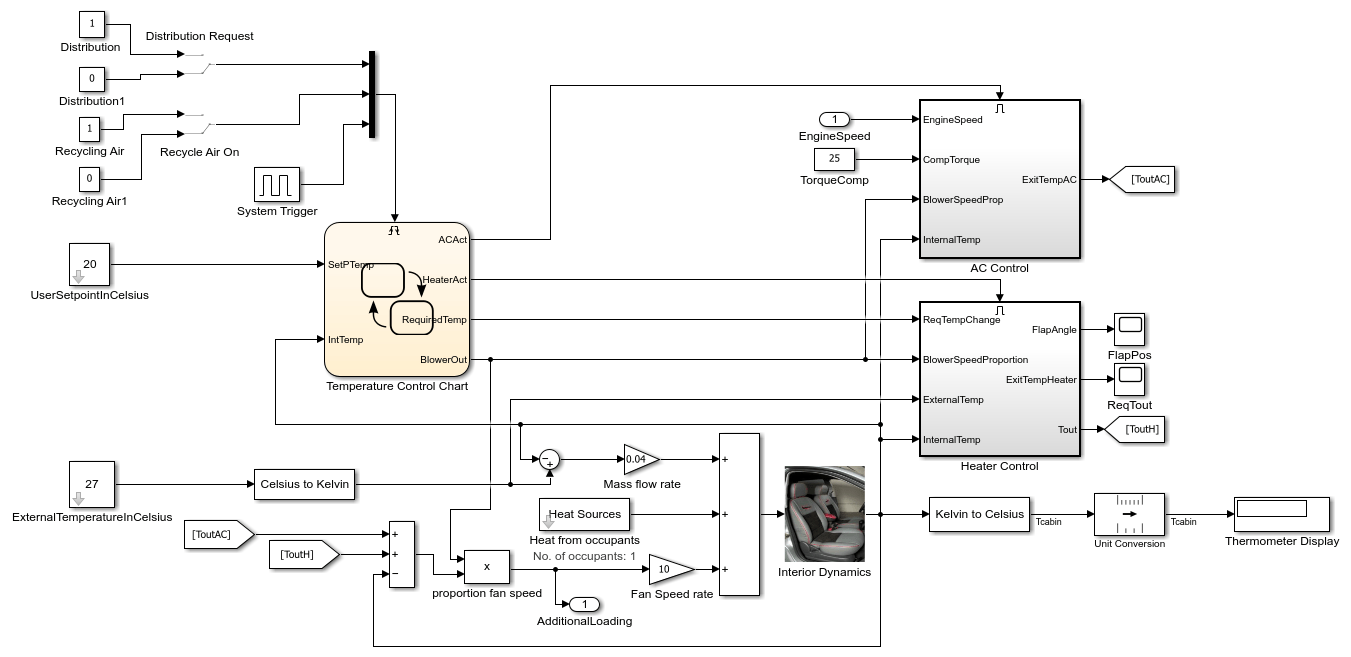### Stateflow® Controller

The control system is implemented in Stateflow®. Double-click the Stateflow chart named Temperature Control to view the supervisory control logic implementation.

The `Heater_AC` state shows that when the setpoint temperature is greater than the current temperature in the vehicle by at least 0.5 degree Celsius, the heater system switches on. The heater remains active until the current internal vehicle temperature is within 0.5 degree Celsius of the setpoint temperature. When the setpoint is at least 0.5 degree Celsius below the current internal vehicle temperature, the air conditioner turns on and stays active until the internal vehicle temperature is within 0.5 degree Celsius of the setpoint temperature. To avoid the problem of continuous switching, the chart implements a 0.5 deg dead band.

In the `Blower` state, the blower output is proportional to the difference between the setpoint temperature and the current temperature. This ensures that the temperature reaches the required value in a reasonable amount of time, despite the temperature difference. When the temperature of the internal vehicle temperature is within 0.5 degree Celsius of the setpoint temperature, the system switches off.

The air distribution, `AirDist`, and recycling air, `Recyc_Air`, are controlled by the two switches that trigger the Stateflow chart. To facilitate window defrosting, the chart implements an internal transition between the two states. When the defrost state is activated, the chart turns off air recycling air.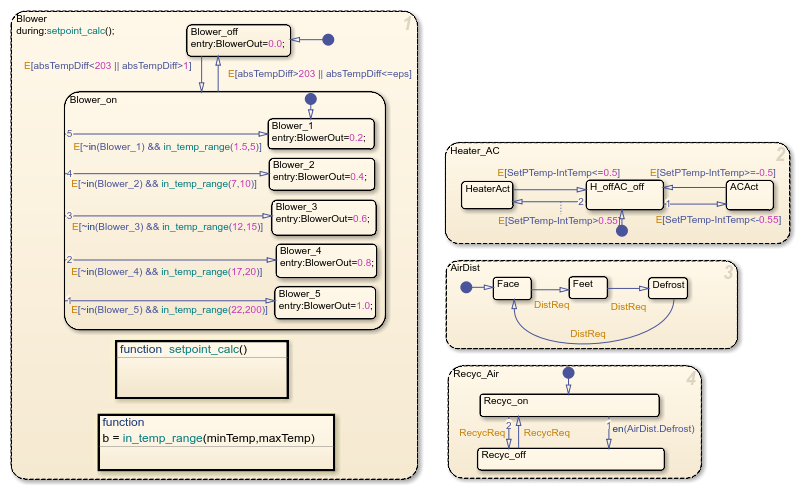### Heater and Air Conditioner Models

The heater model is built from thus equation for a heat exchanger:

`Tout = Ts - (Ts-Tin)e^[(-pi*D*L*hc)/(m_dot*Cp)]`

where:

• Ts = constant (radiator wall temperature)

• D = 0.004m (channel diameter)

• L = 0.05m (radiator thickness)

• N = 30000 (Number of channels)

• k = 0.026 W/mK = constant (thermal conductivity of air)

• Cp = 1007 J/kgK = constant (specific heat of air)

• Laminar flow (hc = 3.66(k/D) = 23.8 W/m2K )

The model considers the effect of the heater flap. Similar to the blower operation, the heat flap output is proportional to the difference between the setpoint temperature and the current temperature. The greater the temperature difference, the more the heat flap opens and increases the heating effect.

The air conditioner (AC) system is one of the two places where the climate control model interfaces with the vehicle electrical system model. The compressor loads the vehicle engine when the AC system is active. The model implements this equation to determine the AC final temperature:

`y*(w*Tcomp) = m_dot*(h4-h1)`

where:

• y = efficiency

• m_dot = mass flow rate

• w = speed of the engine

• Tcomp = compressor torque

• h4, h1 = enthalpy

For the AC system, the model implements bang-bang control. The engine speed and compressor torque control the air temperature that exits the AC.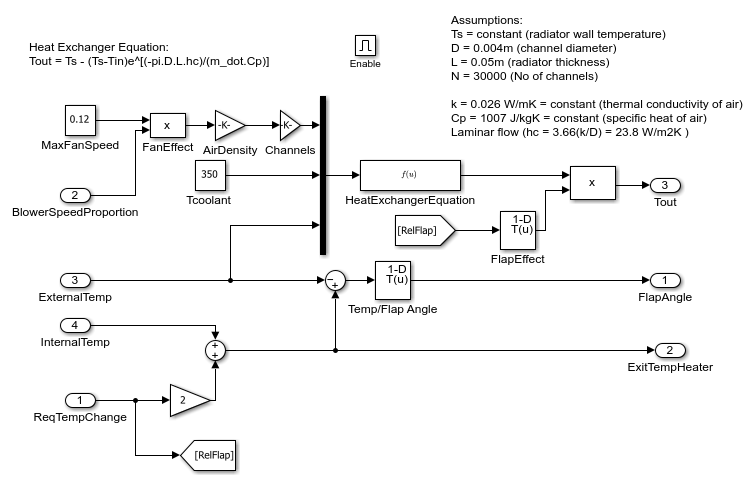### Heat Transfer in Cabin

The temperature of the air felt by the driver is affected by these factors:

• Temperature of the air exiting the vents

• Temperature of the outside air

• Number of people in the vehicle

The value of these factors are inputs into the thermodynamic model of the interior of the cabin. The model accounts for the temperature of the air exiting the vents by calculating the difference between the vent air temperature and the current temperature inside the vehicle and multiplying it by the fan speed proportion (mass flow rate). Then, 100 W of energy is added per person in the vehicle. Lastly, the difference between the temperature of the outside air and the interior air temperature is multiplied by a lesser mass flow rate to account for the air radiating into the vehicle from the outside.

The `Thermometer Display` provides the output of the interior dynamics model. The output is the temperature reading of a sensor behind the head of the driver.

### Electrical System

The electrical system models the vehicle at idle speed. The PID controllers ensure that the vehicle alternator is also operating at the required speed. The alternator is modeled by a synchronous machine that has its field current regulated to control the output voltage. The alternator output is then input to a three-phase six-pulse rectifier bridge to supply the voltage needed to charge the battery. The battery supplies the voltage for the vehicle DC bus.

The DC bus voltage is input to the climate control fan in the climate control, windscreen wipers, and the radio. As the difference between the setpoint temperature and the current vehicle temperature drops, so does the fan speed and loading on the DC bus. The feedback in the electrical system regulates the DC bus voltage.

The additional model of the vehicle electrical system allows for the changing of the engine speed. Changing the engine speed shows the effect on the DC bus voltage.